### Variable resistors

A resistor in an electrical circuit measures the obstruction to current flow. As the name suggests the resistance of a variable resistor can be changed. This allows the current flow through a circuit to be controlled. It can be turned up and down.

The way this is done is fairly simple. A sliding contact on a long coil of wire or length of graphite changes the amount of material in the circuit. The longer the length in the circuit the higher the resistance and the smaller the current.

It might look like this: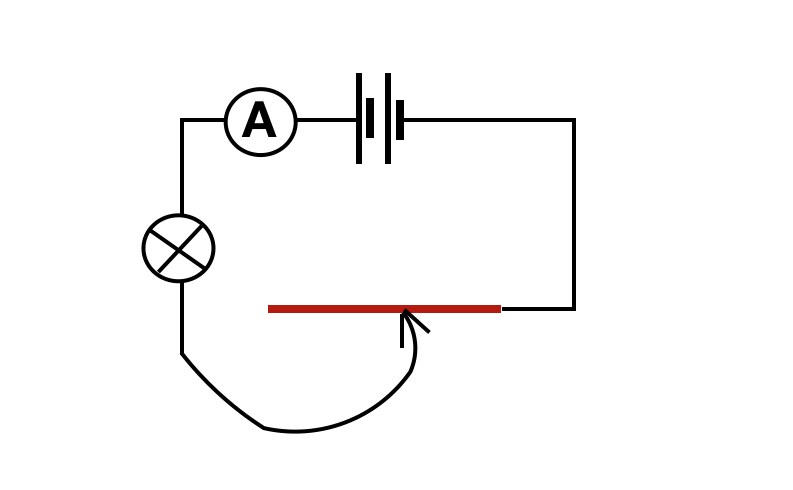Simple variable resistor to control the brightness of a lamp

and a commercial version like this: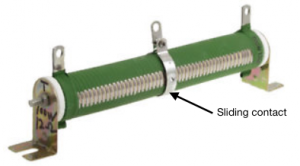Tubular variable resistor

In most cases the resistance material is shaped into a loop so that the whole thing is neater and smaller: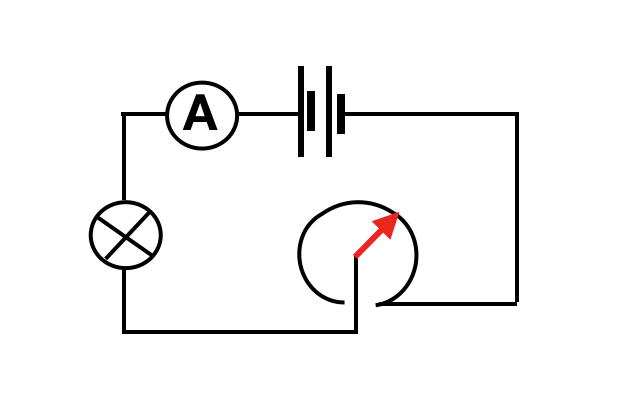If the resistant material, graphite or wire is bent into a loop the device is smaller

The standard circuit symbol is a box (like a fixed resistor) but with an arrow through it: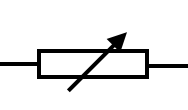Variable resistor circuit symbol

So using it simply to turn the brightness of a bulb up and down the circuit diagram would be: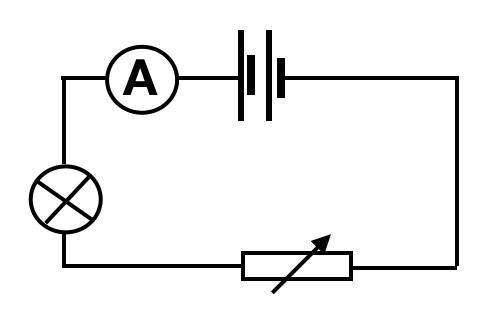Using variable resistor to control the brightness of a lamp